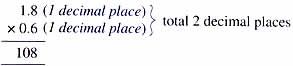Thursday , July 7 2022# NCERT 5th Class (CBSE) Mathematics: Decimals

### B. Multiplying a Decimal by a Decimal

(a) Sapna wants to find out the area of her new carpet. She knows the can find that by multiplying the length and the breadth of the carpet.

1.8 m × 0.6 m = ?

Step 1. Multiply as in whole numbers ignoring the point.

1.9×0.6 = 108

Step 2. Count the number of decimal places in each factor.Step 3. Count off the same number of places from the right to place the decimal point in the product.

1.8×0.6 = 1.08 (2 decimal places)

Answer: The areas of Sapna’s carpet is 1.08 square meters.

(b) 3.1×4.2 = 1302 → 4.2×3.1 13.02

(c) 17.3×1 = 17.3

(d) 14.5×0 = 0

### Zeros in the product

Sometimes you may need to write extra zeros in the product to be able to show the correct number of decimal places.

(a)

## चुनौती हिमालय की 5th NCERT CBSE Hindi Rimjhim Ch 18

चुनौती हिमालय की 5th Class NCERT CBSE Hindi Book Rimjhim Chapter 18 प्रश्न: लद्दाख जम्मू-कश्मीर राज्य में …